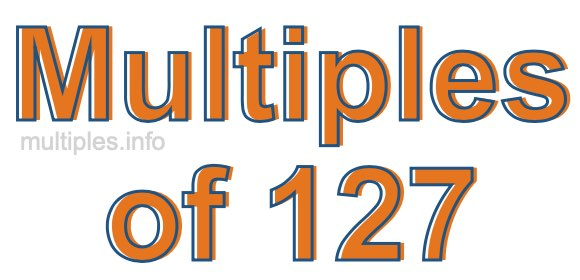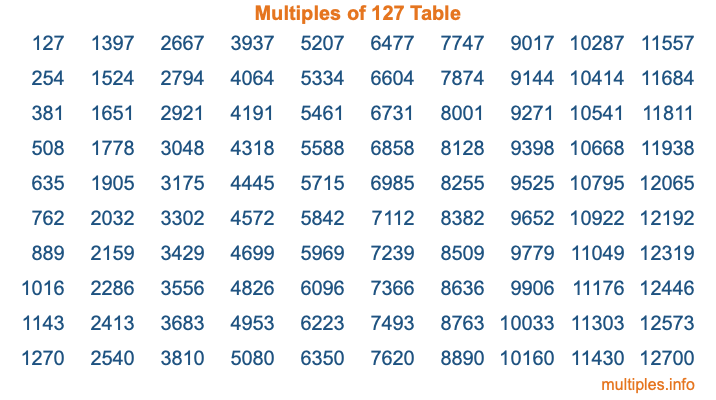Multiples of 127Welcome to the Multiples of 127 page. Here we will first teach you everything you will ever need to know about the multiples of 127, and then give you a study guide summary of everything we taught you to make sure you remember it all. Use this page to look up facts and learn information about the multiples of 127. This page will make you a multiples of one hundred twenty-seven expert!

Definition of Multiples of 127
Multiples of 127 are all the numbers that when divided by 127 equal an integer. Each of the multiples of 127 are called a multiple. A multiple of 127 is created by multiplying 127 by an integer.

Therefore, to create a list of multiples of 127, you start with 1 multiplied by 127, then 2 multiplied by 127, then 3 multiplied by 127, and so on for as long as you want. Thus, the list of the first five multiples of 127 is 127, 254, 381, 508, and 635. To see a larger list of multiples of 127, see the printable image of Multiples of 127 further down on this page. We also have a category where you can choose any nth multiple of 127.

Multiples of 127 Checker
The Multiples of 127 Checker below checks to see if any number of your choice is a multiple of 127. In other words, it checks to see if there is any number (integer) that when multiplied by 127 will equal your number. To do that, we divide your number by 127. If the the quotient is an integer, then your number is a multiple of 127.

Is  a multiple of 127?

Least Common Multiple of 127 and ...
A Least Common Multiple (LCM) is the lowest multiple that two or more numbers have in common. This is also called the smallest common multiple or lowest common multiple and is useful to know when you are adding our subtracting fractions. Enter one or more numbers below (127 is already entered) to find the LCM.

Check out our LCM Calculator if you need more details about the Least Common Multiple or if you need the LCM for different numbers for adding and subtraction fractions.

nth Multiple of 127
As we stated above, 127 is the first multiple of 127, 254 is the second multiple of 127, 381 is the third multiple of 127, and so on. Enter a number below to find the nth multiple of 127.

th multiple of 127

Multiples of 127 vs Factors of 127
127 is a multiple of 127 and a factor of 127, but that is where the similarities end. All postive multiples of 127 are 127 or greater than 127. All positive factors of 127 are 127 or less than 127.

Below is the beginning list of multiples of 127 and the factors of 127 so you can compare:

Multiples of 127: 127, 254, 381, 508, 635, etc.

Factors of 127: 1, 127

As you can see, the multiples of 127 are all the numbers that you can divide by 127 to get a whole number. The factors of 127, on the other hand, are all the whole numbers that you can multiply by another whole number to get 127.

It's also interesting to note that if a number (x) is a factor of 127, then 127 will also be a multiple of that number (x).

Multiples of 127 vs Divisors of 127
The divisors of 127 are all the integers that 127 can be divided by evenly. Below is a list of the divisors of 127.

Divisors of 127: 1, 127

The interesting thing to note here is that if you take any multiple of 127 and divide it by a divisor of 127, you will see that the quotient is an integer.

Multiples of 127 Table
Below is an image of the first 100 multiples of 127 in a table. The table is in chronological order, column by column. The first column has the first ten multiples of 127, the second column has the next ten multiples of 127, and so on.The Multiples of 127 Table is also referred to as the 127 Times Table or Times Table of 127. You are welcome to print out our table for your studies.

Negative Multiples of 127
Although not often discussed or needed in math, it is worth mentioning that you can make a list of negative multiples of 127 by multiplying 127 by -1, then by -2, then by -3, and so on, to get the following list of negative multiples of 127:

-127, -254, -381, -508, -635, etc.

Multiples of 127 Summary
Below is a summary of important Multiples of 127 facts that we have discussed on this page. To retain the knowledge on this page, we recommend that you read through the summary and explain to yourself or a study partner why they hold true.

There are an infinite number of multiples of 127.

A multiple of 127 divided by 127 will equal a whole number.

127 divided by a factor of 127 equals a divisor of 127.

The nth multiple of 127 is n times 127.

The largest factor of 127 is equal to the first positive multiple of 127.

127 is a multiple of every factor of 127.

127 is a multiple of 127.

A multiple of 127 divided by a divisor of 127 equals an integer.

127 divided by a divisor of 127 equals a factor of 127.

Any integer times 127 will equal a multiple of 127.

Multiples of a Number
Here you can get the multiples of another number, all with the same attention to detail as we did for multiples of 127 on this page.

Multiples of
Multiples of 128
Did you find our page about multiples of one hundred twenty-seven educational? Do you want more knowledge? Check out the multiples of the next number on our list!